## Control Structures

OptoScript provides the following control structures to control the flow of logic in the code:

• If Statements
• Switch or Case Statements
• While Loops
• Repeat Loops
• For Loops

### If Statements

If statements offer branching in logic:

• if statement A is true, then one action is taken;
• if statement A is false, a different action is taken.

If statements are very flexible. Here are several examples of how you can use them: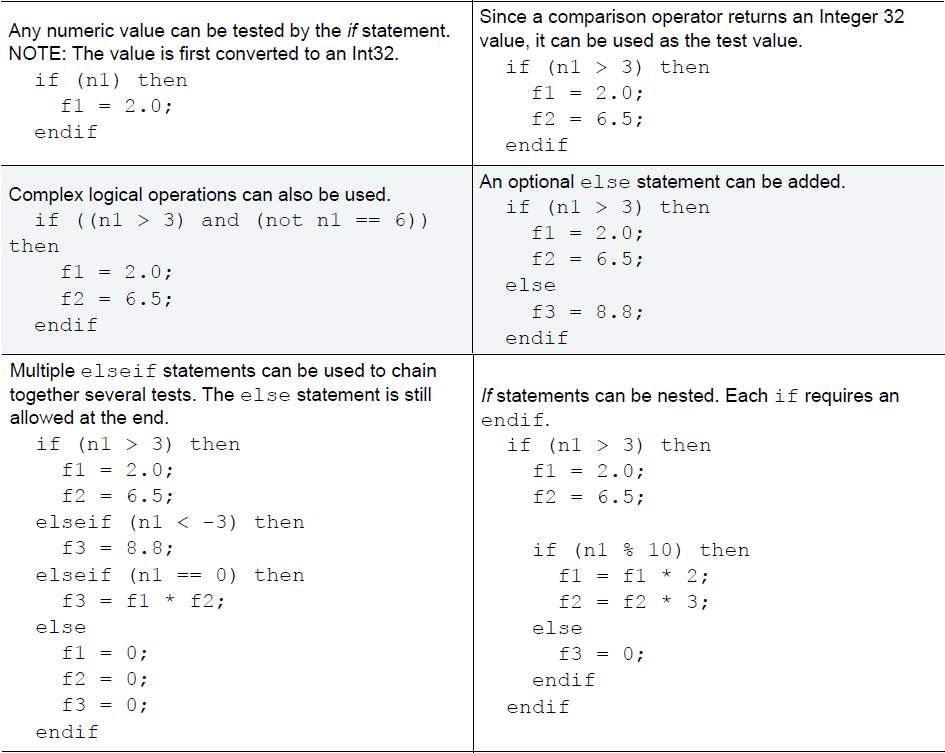### Switch or Case Statements

A switch or case statement offers branching logic and can be used in place of if statements when the expression can match one of several numeric values with these considerations:

• The value for each case can only be a numeric constant or a mathematical expression.
• Comparisons, logical operators, and strings can’t be used in cases.
• If a case involves a float, the float is converted to an integer before it’s used.
• Only one case can be tested at a time, as seen in these examples.

Here's an example of a switch statement: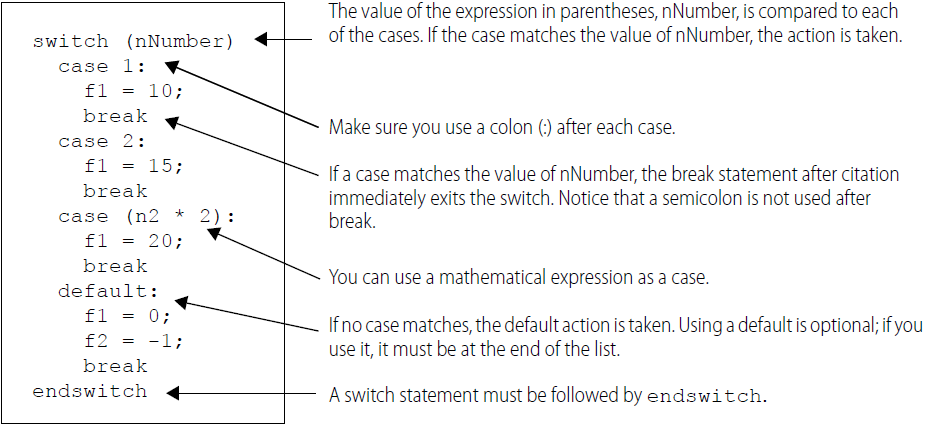### While Loops

The while loop is used to execute a list of statements while a given condition is true. The condition is tested at the beginning of each loop.

For example, this loop sets the first five elements (elements 0 through 4) of a table (ntTable) to a value of 10: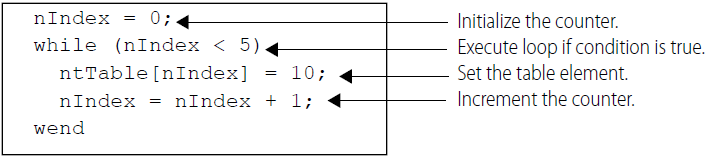While loops can be nested and can contain other kinds of programming statements. Each while needs a matching wend at the end.

For example: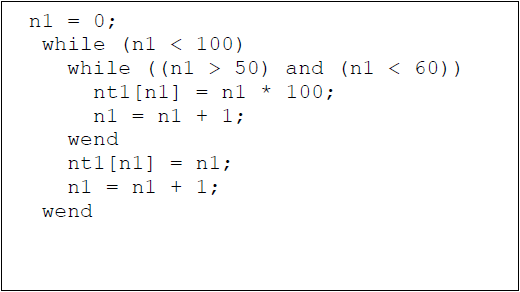### Repeat Loops

Repeat loops, in contrast to while loops, are used to execute a list of statements until a given condition is true. Because the condition is tested at the end of each loop, the contents of the loop will always be executed at least once.

This example sets the first five elements of ntTable to 10. Do you see the difference compared to the while loop? The test for the condition is at the end of the loop, after until (nIndex >= 5).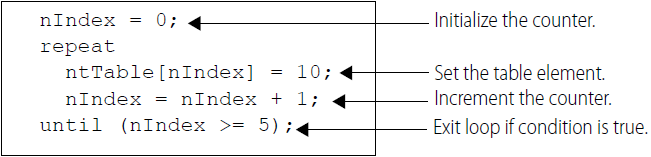Repeat loops can be nested and can contain other kinds of programming statements. Each repeat statement needs a matching until statement at the end.

### For Loops

For loops can be used to execute a list of statements a certain number of times.

The for line sets up the initial value and the predefined final value for the counter that counts the repetitions. The line also includes the steps by which the counter increments from its initial value, to its final value (Ex: step 1 counts by ones; step 2 counts by twos, and so on). The step is required. The counter can be any numeric variable or I/O point, but its value will always be a whole number (Integer 32). The initial value, final value, and step can be any numeric expression, just remember they are always converted to an Integer 32 value.

CAUTION: A step value of zero creates an infinite loop. A float step value between –0.5 and 0.5 also creates an infinite loop, since it is rounded to zero when converted to an integer 32.

This example results in nVariable equaling 6: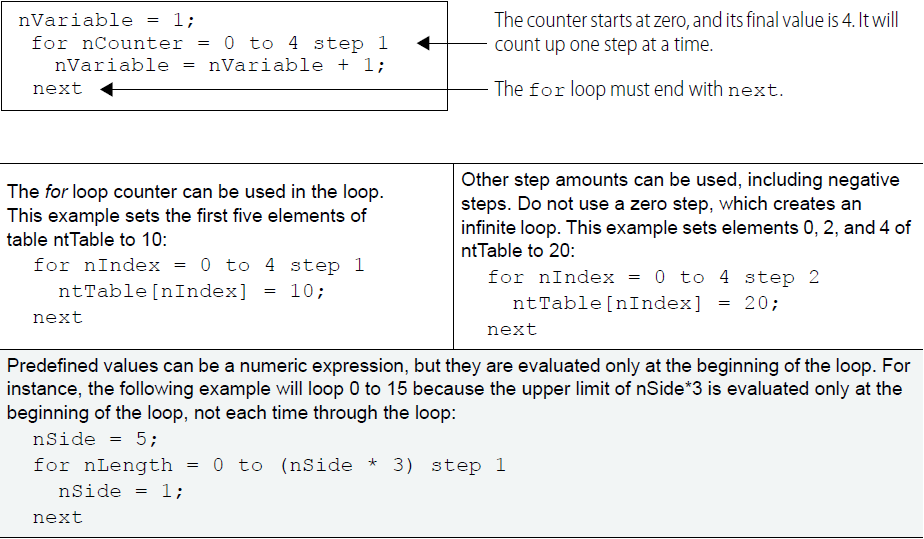For loops can be nested and can contain other types of statements. Each for requires a next at the end of the loop.

#### Summary

Learn about the control structures available in OptoScript to control the logic flow:

• If Statements
• Switch or Case Statements
• While Loops
• Repeat Loops
• For Loops